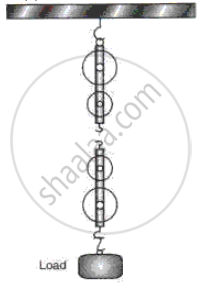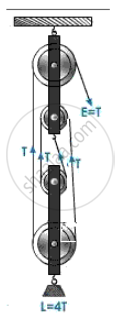Advertisement Remove all ads

# (A) Fig Represents an Incomplete Diagram of a Simple String Pulley System. - Physics

Numerical

(a)  Fig represents an incomplete diagram of a simple string pulley      system. Copy this diagram on a new page and complete it and mark where the effort must be applied to lift the load.
(b) What is the velocity ratio of this system?
(c) If the pulley system is 80% efficient and the load is 720 N, then
(i) What effort must be applied to lift the load?
(ii) What work must be done must be done in lifting the load through   a distance of 2 m using this machine?Advertisement Remove all ads

#### Solution

a) Corrert wínding ofrope around pulleys, load and effortis as shownb) Since there are four strings in the block and tackle system, therefore, the velocíty ratio ofthe systemís V.R = n = 4

c)

(i) Given load L = 720 N

Let effort = E

Efficiency = "M.A"/"V.R"

or, 80% = "L/E"/4

or, 80/100 xx 4 = 720/E

or, E = (720 xx 100)/(80 xx 4) = 225 N

(ii) Given, Load L = 720 N

Displacement - of - load, dL = 2m

Efficiency = "work - output"/"work - input"

or work - input = "work - output"/"efficiency" = ("Load" xx "d"_"L")/"n"

or, Work - input = (720 xx 2)/0.8 = 1800 J

Is there an error in this question or solution?
Advertisement Remove all ads

#### APPEARS IN

Frank ICSE Class 10 Physics Part 2
Chapter 1 Force, Work, Energy and Power
Exercise | Q 4 | Page 61
Advertisement Remove all ads
Advertisement Remove all ads
Share
Notifications

View all notifications

Forgot password?
Course